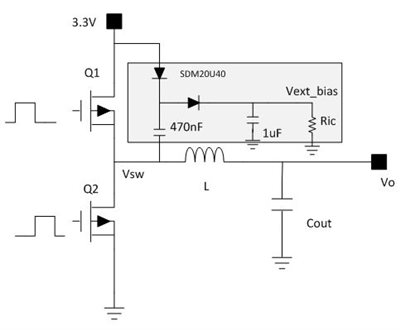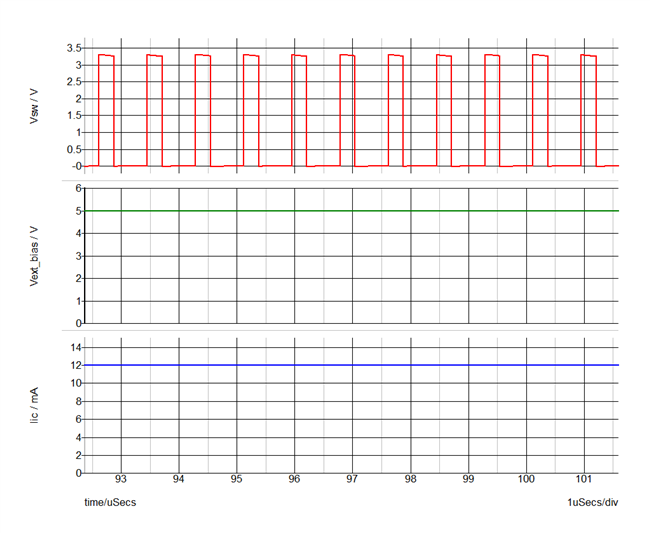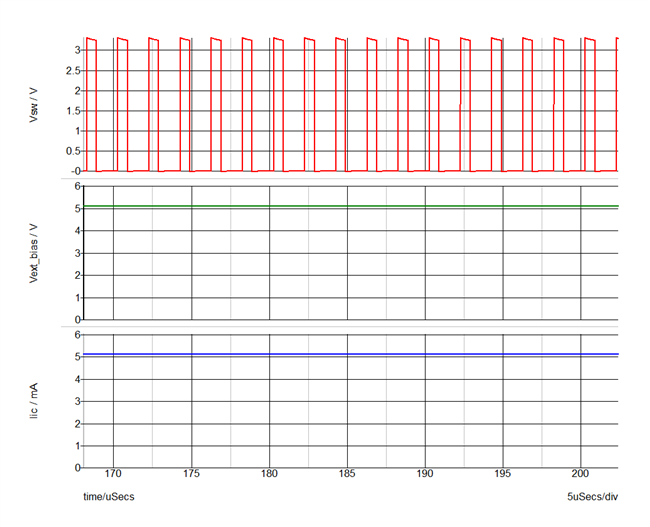# When and how to supply an external bias for buck controllers – part 3

In part 1 of this series, I discussed the need for an external bias and under what conditions you need to consider one. In part 2, I discussed whether you can apply an external bias to any controller. In this third and final installment, I will go over a circuit that you can use to generate an external bias for any controller. This may prove useful in cases where a 5V bias rail is not available for providing the external bias.

Looking at Figure 1, the shaded region is the external circuit for the bias. Ric represents the current drawn by the integrated circuit (IC).Figure 1: External bias circuit shown in the shaded box

You must make sure that the external bias circuit can provide the current, since it depends on the operating frequency, selected field-effect transistors (FETs), etc.

Iic = (Qg(Q1) + Qg(Q2))*1e-09*Fsw*1e03                              (1)

Let’s plug in some numbers and use the example of a device running at two different frequencies and using the TI CSD87381P NexFET™ power block. These are the simulation conditions:

• Qg(Q1) = 3.9nC.
• Qg(Q2) = 6.2nC.
• Iic = 12mA at 1,200kHz.
• Iic = 5mA at 500kHz.

Once you figure out Iic, you can calculate Ric for the simulations by Vext_bias/Iic.

Run the simulations with the calculated Ric and make sure that Vext_bias does not exceed the absolute maximum of the VDD pin, or the pin of the IC where you need to apply the external bias. In some cases, you may need a Zener diode or additional loading resistors.

Figures 2 and 3 show the circuit waveforms. You can see that I generated close to 5V for the external bias. As the efficiency curves in part 1 of this series illustrated, this approach helps increase efficency or enables circuits to support higher currents, whatever the case may be.Figure 2: Waveforms at Fsw = 1,200kHz at Iic = 12mAFigure 3: Waveforms at Fsw = 500kHz at Iic = 5mA

There are many charge-pump schemes possible with various variants and I have demonstrated a simple method to implement an external bias. One point to note is the variation of the 3.3V rail. If the 3.3V rail has a wide tolerance, I recommend placing a Zener diode to clamp the voltage applied to the VDD pin in case of overshoot on the input voltage rail.

Thank you for reading this series of blogs. I hope you learned when and how to supply an external bias for buck controllers. Feel free to post a comment if you have any questions.